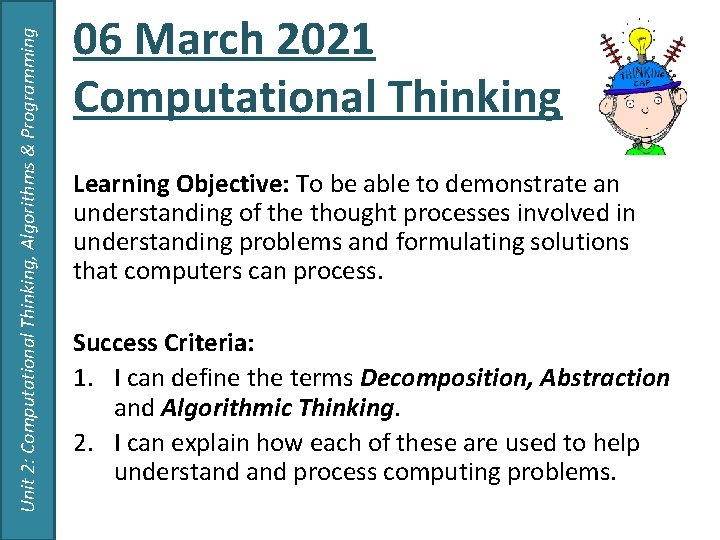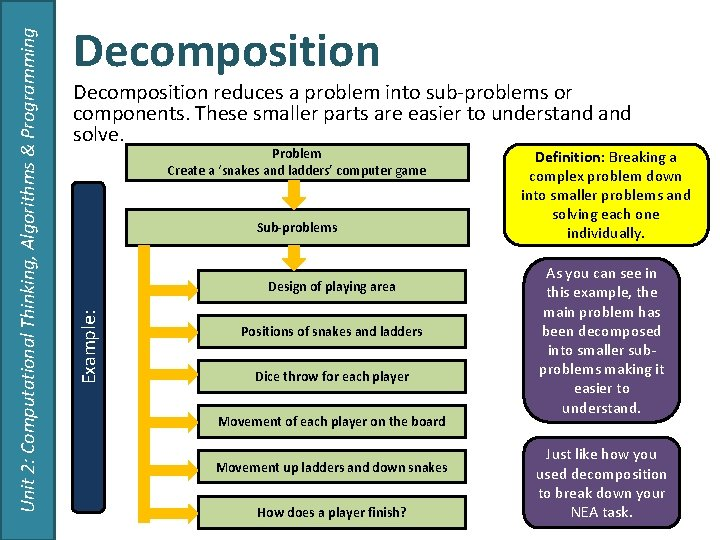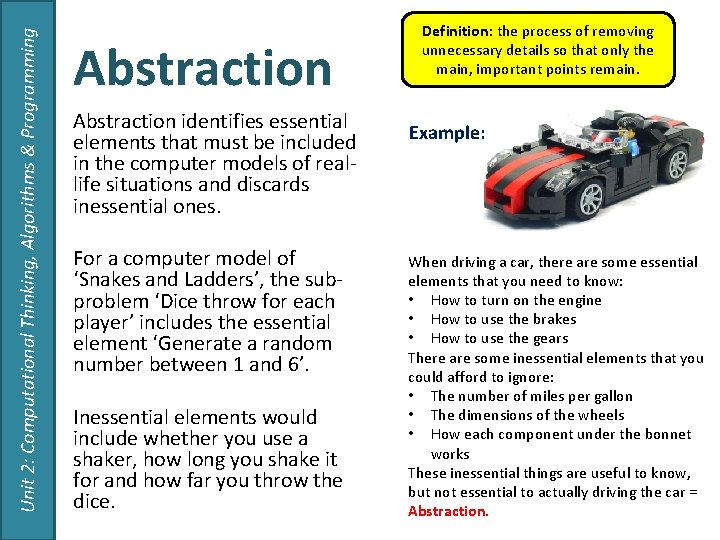# Unit 2 Computational Thinking Algorithms Programming Computational Thinking

• Slides: 5Unit 2: Computational Thinking, Algorithms & Programming Computational Thinking 2. 1 - AlgorithmsUnit 2: Computational Thinking, Algorithms & Programming 06 March 2021 Computational Thinking Learning Objective: To be able to demonstrate an understanding of the thought processes involved in understanding problems and formulating solutions that computers can process. Success Criteria: 1. I can define the terms Decomposition, Abstraction and Algorithmic Thinking. 2. I can explain how each of these are used to help understand process computing problems.Decomposition reduces a problem into sub-problems or components. These smaller parts are easier to understand solve. Problem Create a ‘snakes and ladders’ computer game Sub-problems Design of playing area Example: Unit 2: Computational Thinking, Algorithms & Programming Decomposition Positions of snakes and ladders Dice throw for each player Movement of each player on the board Movement up ladders and down snakes How does a player finish? Definition: Breaking a complex problem down into smaller problems and solving each one individually. As you can see in this example, the main problem has been decomposed into smaller subproblems making it easier to understand. Just like how you used decomposition to break down your NEA task.Unit 2: Computational Thinking, Algorithms & Programming Abstraction identifies essential elements that must be included in the computer models of reallife situations and discards inessential ones. For a computer model of ‘Snakes and Ladders’, the subproblem ‘Dice throw for each player’ includes the essential element ‘Generate a random number between 1 and 6’. Inessential elements would include whether you use a shaker, how long you shake it for and how far you throw the dice. Definition: the process of removing unnecessary details so that only the main, important points remain. Example: When driving a car, there are some essential elements that you need to know: • How to turn on the engine • How to use the brakes • How to use the gears There are some inessential elements that you could afford to ignore: • The number of miles per gallon • The dimensions of the wheels • How each component under the bonnet works These inessential things are useful to know, but not essential to actually driving the car = Abstraction.Unit 2: Computational Thinking, Algorithms & Programming Algorithmic Thinking Algorithmic thinking is a subset of computational thinking that involves defining a clear set of instructions to solve a problem. Once a successful solution to a problem has been found, it can be used repeatedly for the same problem. For example, the process of calculating the mean of a set of numbers is always the same irrespective of how many numbers and what they are – add up all the numbers and divide the total by the number in the set. Definition: a logical way of getting from the problem to the solution. A set of instructions for solving a problem.